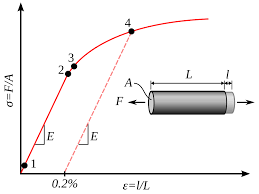## How to Calculate and Solve for Plastic Pressure | Polymer & TextileThe image above represents plastic pressure.

To compute for plastic pressure, two essential parameters are needed and these parameters are hydraulic pressure (PH) and intensification ratio (Ir).

The formula for calculating plastic pressure:

Pp = PH x Ir

Where;

Pp = Plastic Pressure
PH = Hydraulic Pressure
Ir = Intensification Ratio

Let’s solve an example;
Find the plastic pressure when the hydraulic pressure is 20 and the intensification ratio is 44.

This implies that;

PH = Hydraulic Pressure = 20
Ir = Intensification Ratio = 44

Pp = PH x Ir
Pp = 20 x 44
Pp = 880

Therefore, the plastic pressure is 880.

Calculating the Hydraulic Pressure when the Plastic Pressure and the Intensification Ratio is Given.

PH = Pp / Ir

Where;

PH = Hydraulic Pressure
Pp = Plastic Pressure
Ir = Intensification Ratio

Let’s solve an example;
Find the hydraulic pressure when the plastic pressure is 24 and the intensification ratio is 10.

This implies that;

Pp = Plastic Pressure = 24
Ir = Intensification Ratio = 10

PH = Pp / Ir
PH = 24 / 10
PH = 2.4

Therefore, the hydraulic pressure is 2.4.
Continue reading How to Calculate and Solve for Plastic Pressure | Polymer & Textile